Скачать презентацию 529 Chapter 15 REAL ESTATE MATH 532

• Количество слайдов: 46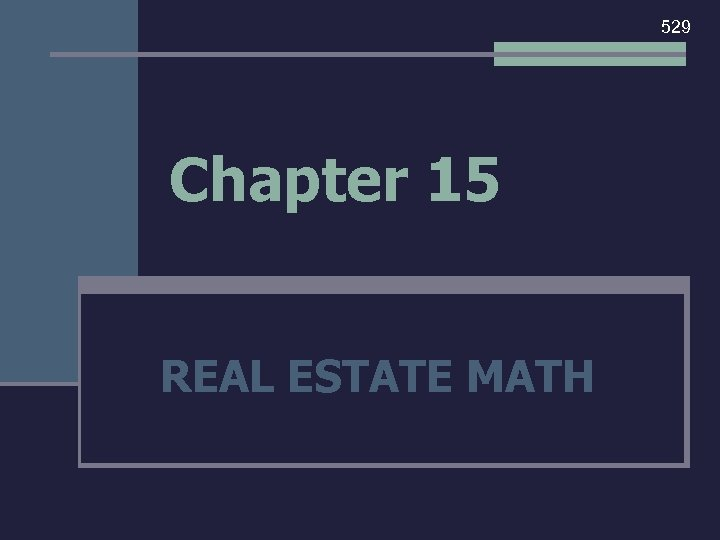529 Chapter 15 REAL ESTATE MATH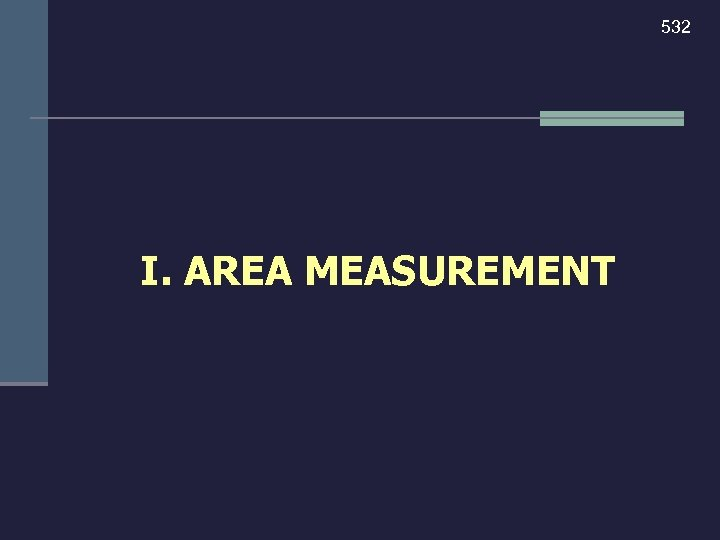532 I. AREA MEASUREMENT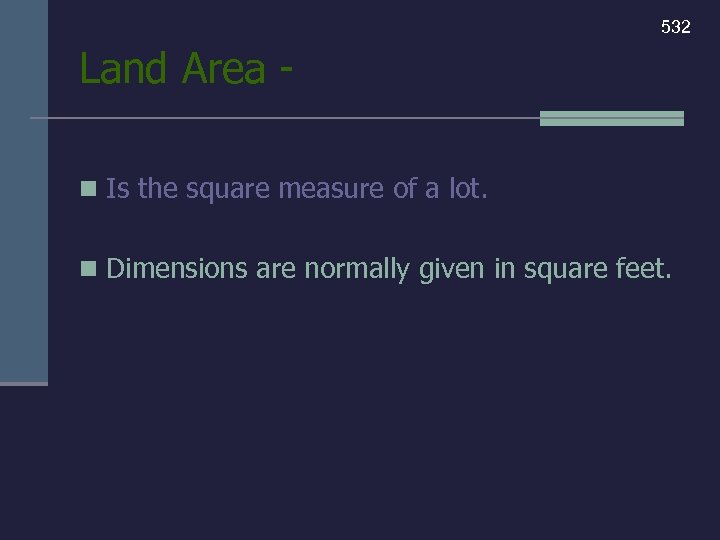532 Land Area n Is the square measure of a lot. n Dimensions are normally given in square feet.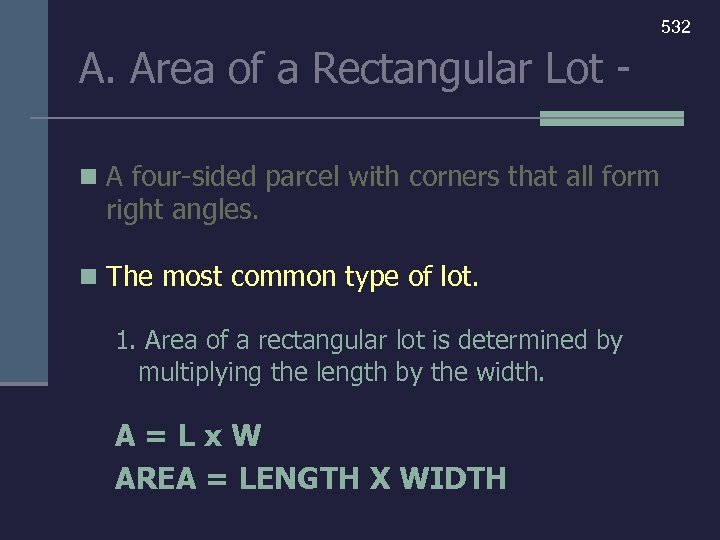532 A. Area of a Rectangular Lot n A four-sided parcel with corners that all form right angles. n The most common type of lot. 1. Area of a rectangular lot is determined by multiplying the length by the width. A=Lx. W AREA = LENGTH X WIDTH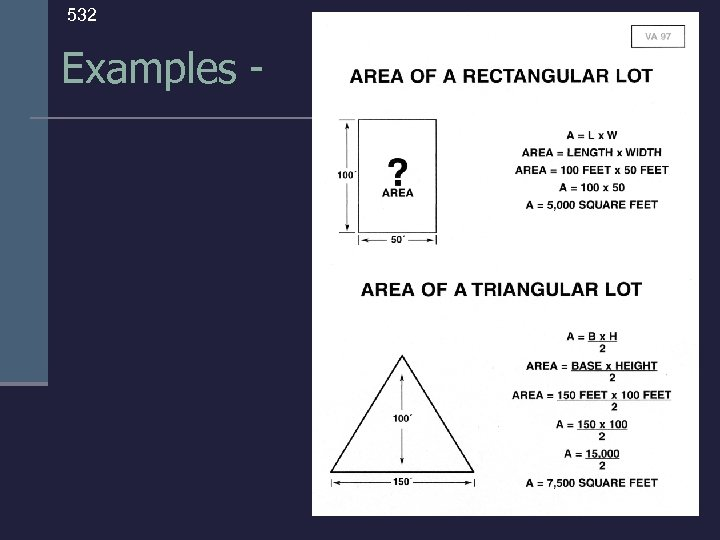532 Examples -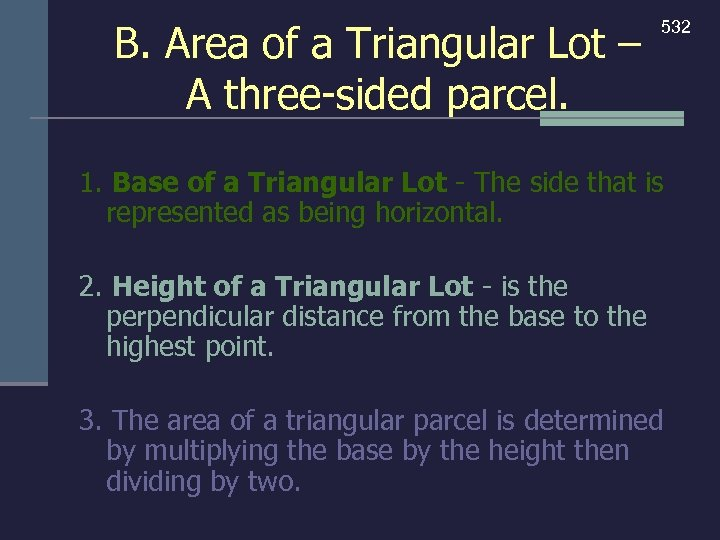B. Area of a Triangular Lot – A three-sided parcel. 532 1. Base of a Triangular Lot - The side that is represented as being horizontal. 2. Height of a Triangular Lot - is the perpendicular distance from the base to the highest point. 3. The area of a triangular parcel is determined by multiplying the base by the height then dividing by two.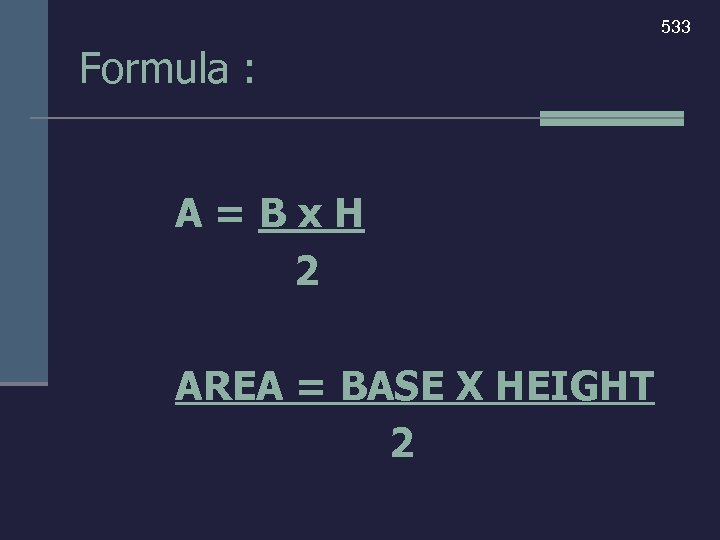533 Formula : A=Bx. H 2 AREA = BASE X HEIGHT 2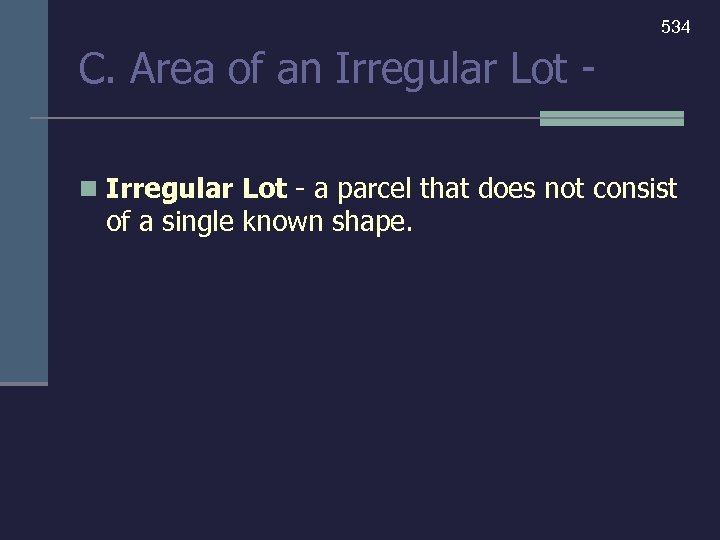534 C. Area of an Irregular Lot - a parcel that does not consist of a single known shape.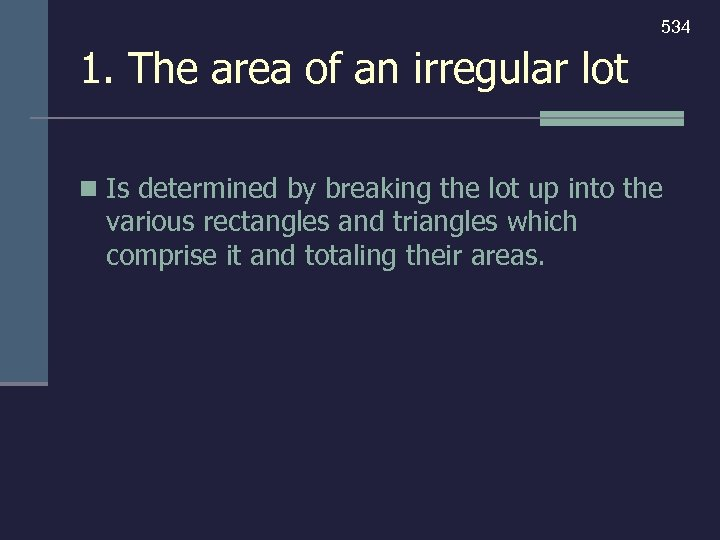534 1. The area of an irregular lot n Is determined by breaking the lot up into the various rectangles and triangles which comprise it and totaling their areas.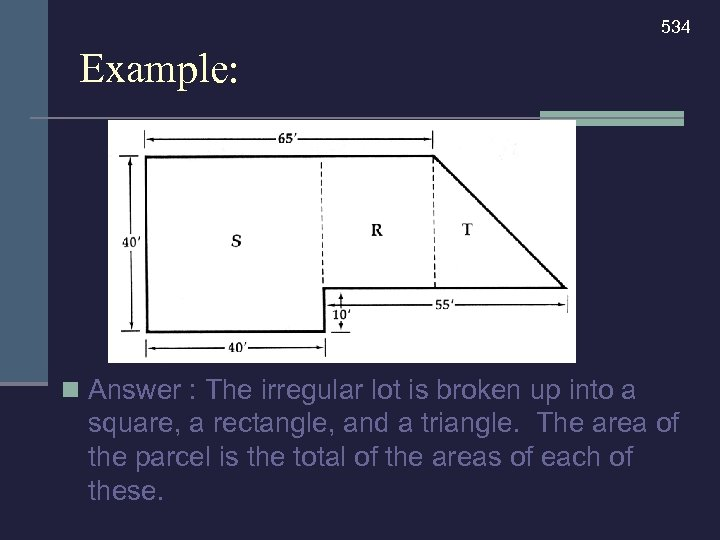534 Example: n Answer : The irregular lot is broken up into a square, a rectangle, and a triangle. The area of the parcel is the total of the areas of each of these.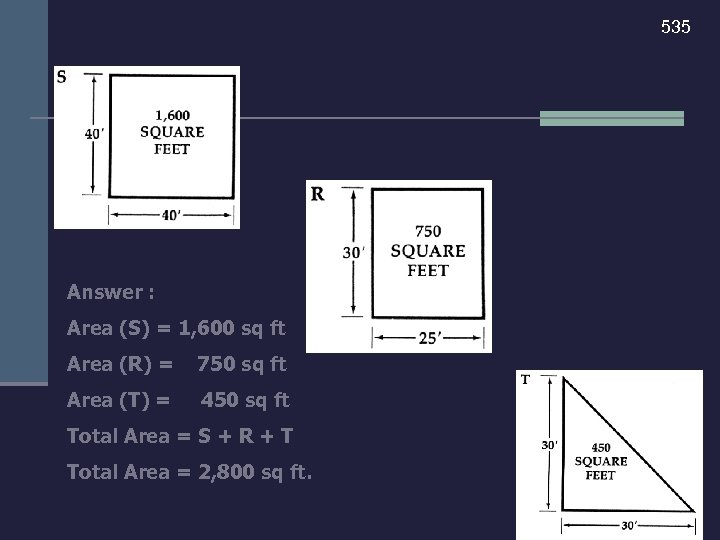535 Answer : Area (S) = 1, 600 sq ft Area (R) = 750 sq ft Area (T) = 450 sq ft Total Area = S + R + T Total Area = 2, 800 sq ft.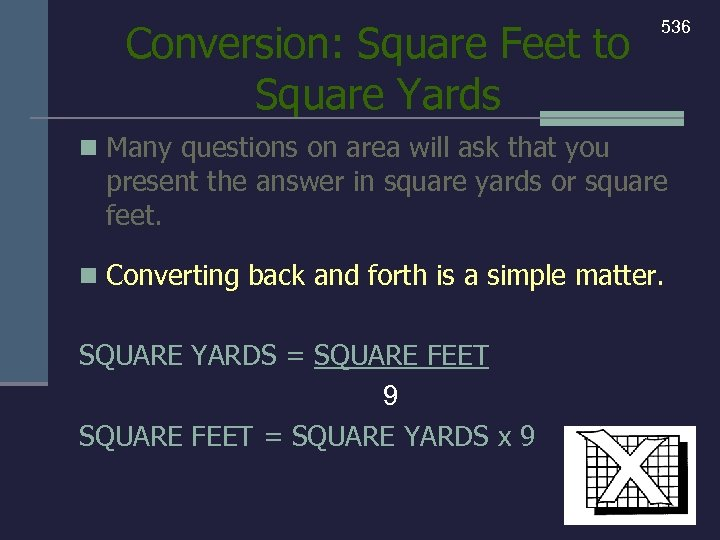Conversion: Square Feet to Square Yards 536 n Many questions on area will ask that you present the answer in square yards or square feet. n Converting back and forth is a simple matter. SQUARE YARDS = SQUARE FEET 9 SQUARE FEET = SQUARE YARDS x 9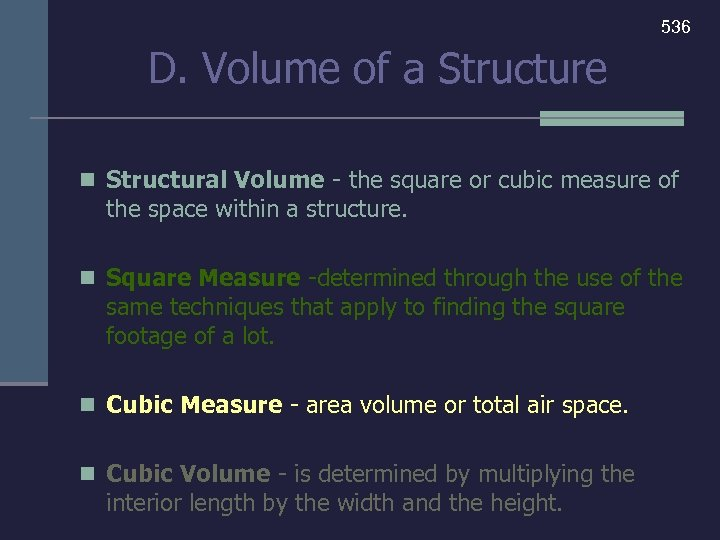536 D. Volume of a Structure n Structural Volume - the square or cubic measure of the space within a structure. n Square Measure -determined through the use of the same techniques that apply to finding the square footage of a lot. n Cubic Measure - area volume or total air space. n Cubic Volume - is determined by multiplying the interior length by the width and the height.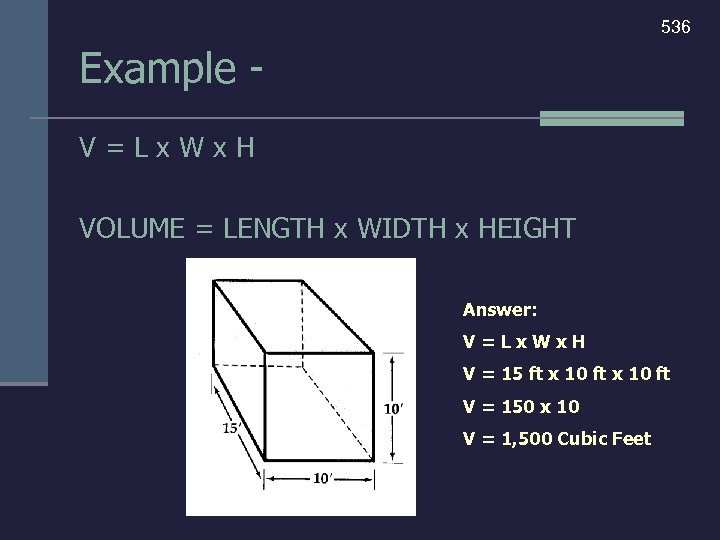536 Example V=Lx. Wx. H VOLUME = LENGTH x WIDTH x HEIGHT Answer: V=Lx. Wx. H V = 15 ft x 10 ft V = 150 x 10 V = 1, 500 Cubic Feet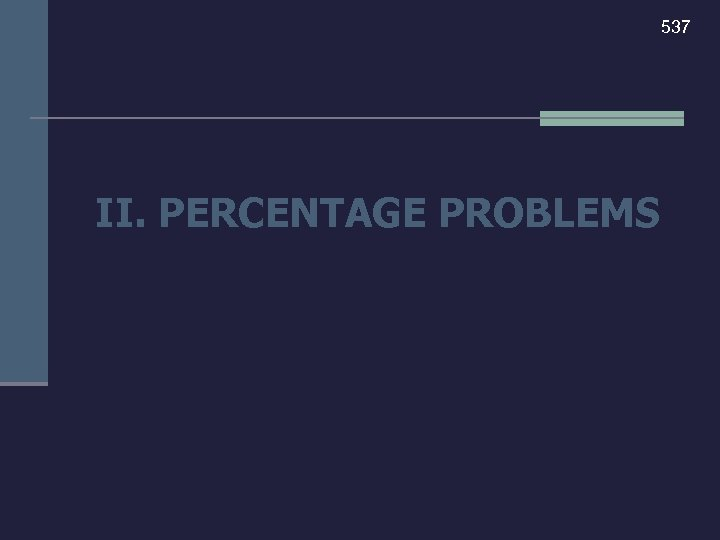537 II. PERCENTAGE PROBLEMS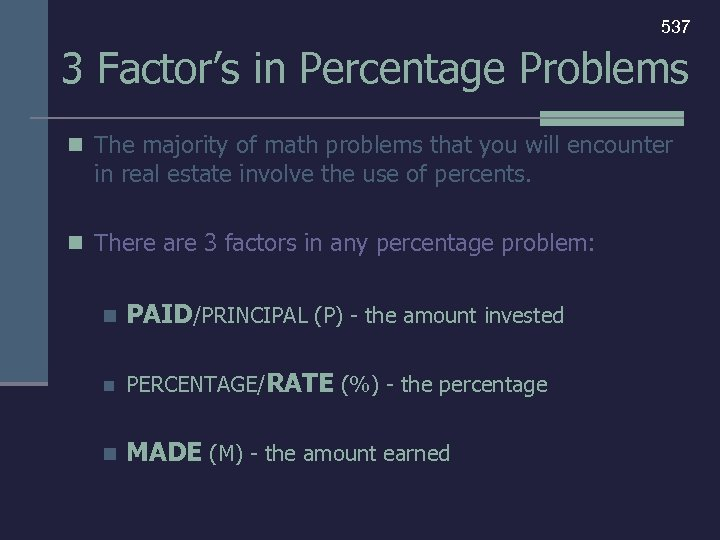537 3 Factor’s in Percentage Problems n The majority of math problems that you will encounter in real estate involve the use of percents. n There are 3 factors in any percentage problem: n PAID/PRINCIPAL (P) - the amount invested n PERCENTAGE/RATE (%) - the percentage n MADE (M) - the amount earned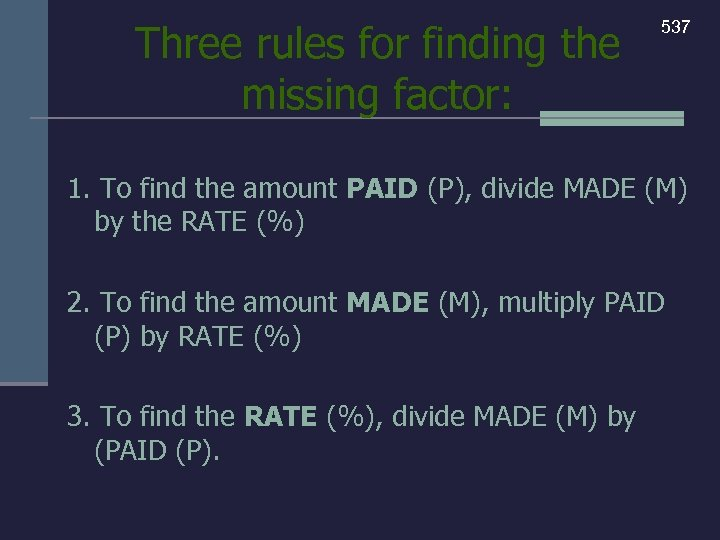Three rules for finding the missing factor: 537 1. To find the amount PAID (P), divide MADE (M) by the RATE (%) 2. To find the amount MADE (M), multiply PAID (P) by RATE (%) 3. To find the RATE (%), divide MADE (M) by (PAID (P).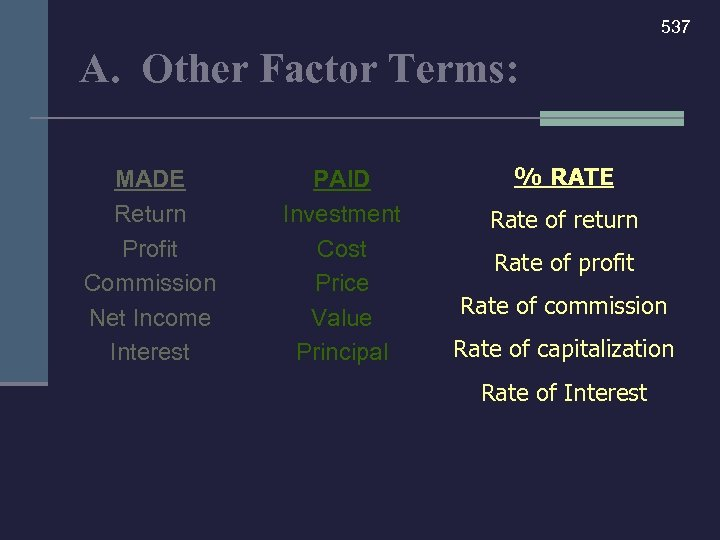537 A. Other Factor Terms: MADE Return Profit Commission Net Income Interest PAID Investment Cost Price Value Principal % RATE Rate of return Rate of profit Rate of commission Rate of capitalization Rate of Interest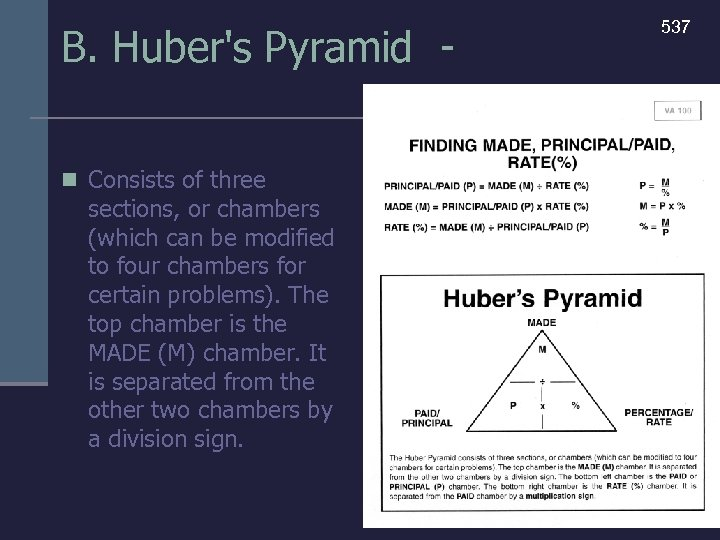B. Huber's Pyramid n Consists of three sections, or chambers (which can be modified to four chambers for certain problems). The top chamber is the MADE (M) chamber. It is separated from the other two chambers by a division sign. 537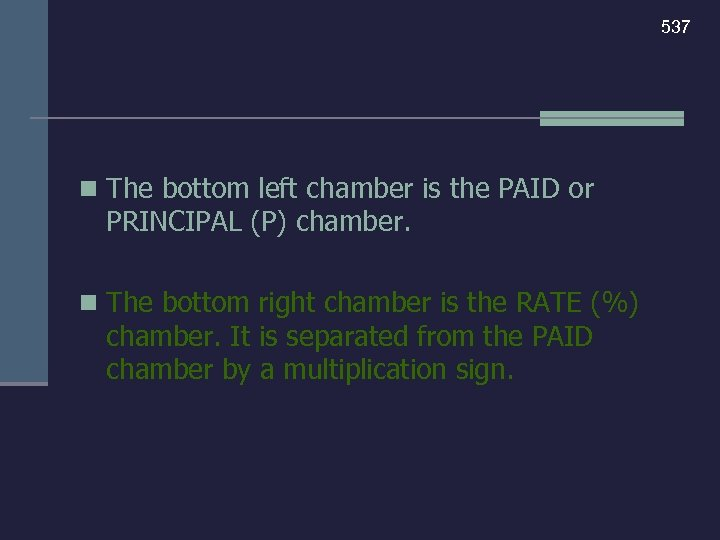537 n The bottom left chamber is the PAID or PRINCIPAL (P) chamber. n The bottom right chamber is the RATE (%) chamber. It is separated from the PAID chamber by a multiplication sign.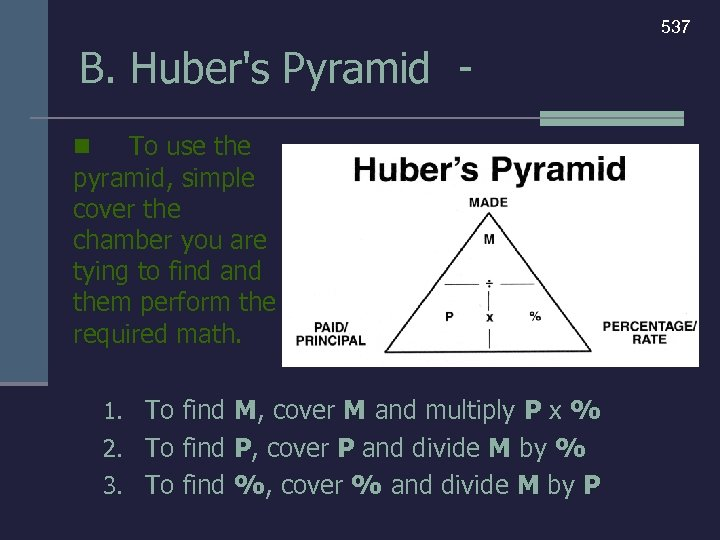537 B. Huber's Pyramid To use the pyramid, simple cover the chamber you are tying to find and them perform the required math. n 1. To find M, cover M and multiply P x % 2. To find P, cover P and divide M by % 3. To find %, cover % and divide M by P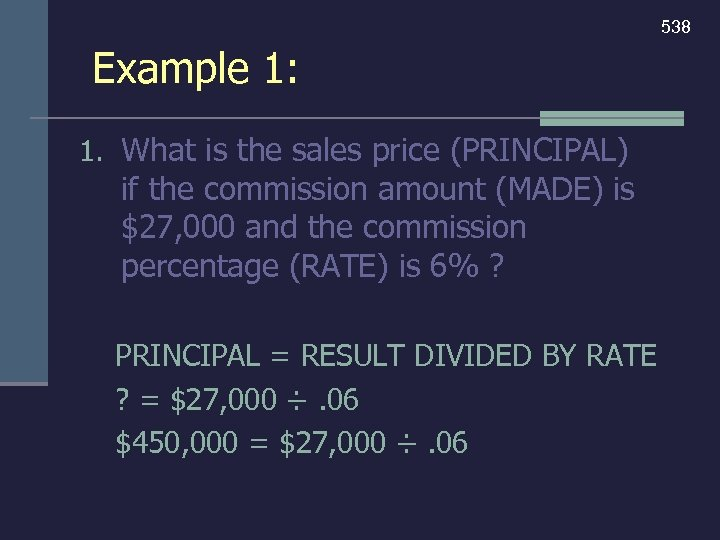538 Example 1: 1. What is the sales price (PRINCIPAL) if the commission amount (MADE) is \$27, 000 and the commission percentage (RATE) is 6% ? PRINCIPAL = RESULT DIVIDED BY RATE ? = \$27, 000 ÷. 06 \$450, 000 = \$27, 000 ÷. 06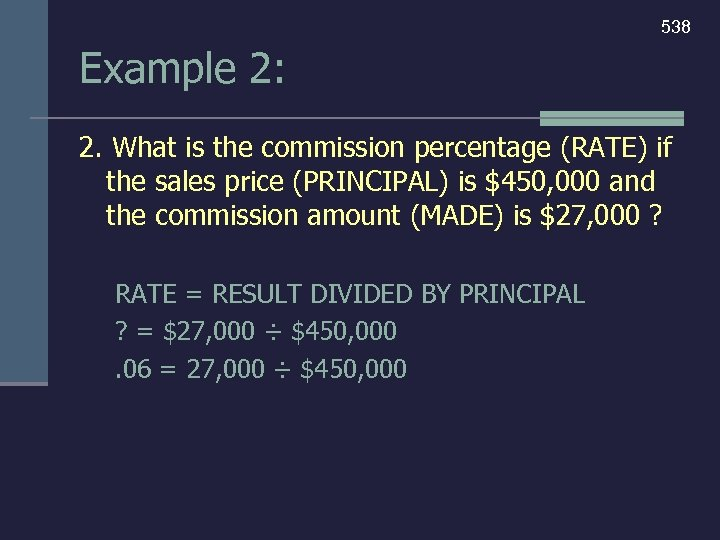538 Example 2: 2. What is the commission percentage (RATE) if the sales price (PRINCIPAL) is \$450, 000 and the commission amount (MADE) is \$27, 000 ? RATE = RESULT DIVIDED BY PRINCIPAL ? = \$27, 000 ÷ \$450, 000. 06 = 27, 000 ÷ \$450, 000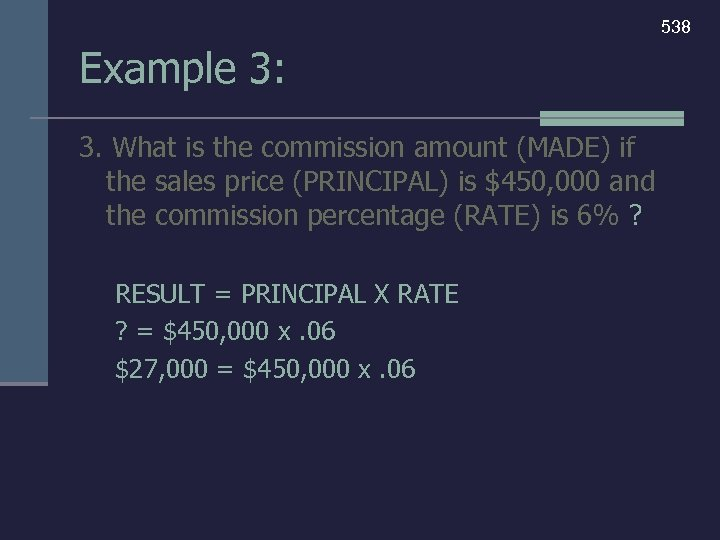538 Example 3: 3. What is the commission amount (MADE) if the sales price (PRINCIPAL) is \$450, 000 and the commission percentage (RATE) is 6% ? RESULT = PRINCIPAL X RATE ? = \$450, 000 x. 06 \$27, 000 = \$450, 000 x. 06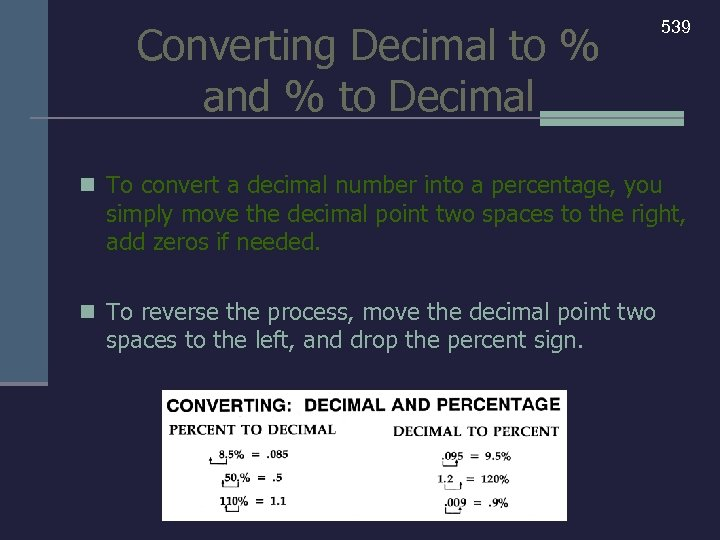Converting Decimal to % and % to Decimal 539 n To convert a decimal number into a percentage, you simply move the decimal point two spaces to the right, add zeros if needed. n To reverse the process, move the decimal point two spaces to the left, and drop the percent sign.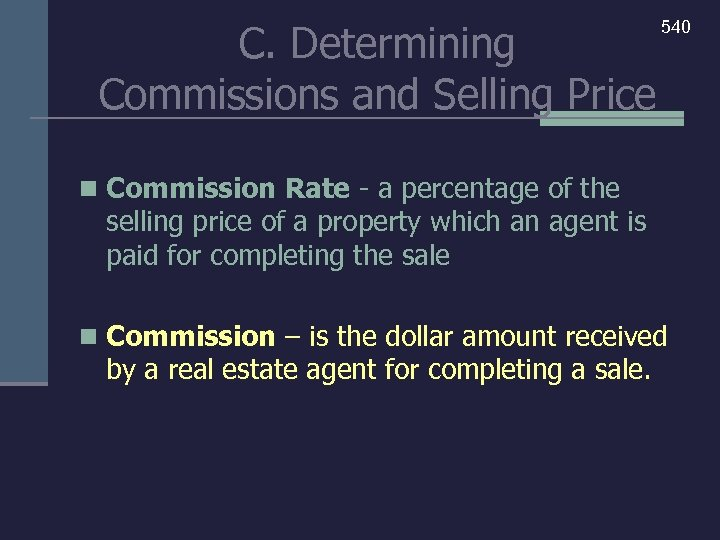C. Determining Commissions and Selling Price 540 n Commission Rate - a percentage of the selling price of a property which an agent is paid for completing the sale n Commission – is the dollar amount received by a real estate agent for completing a sale.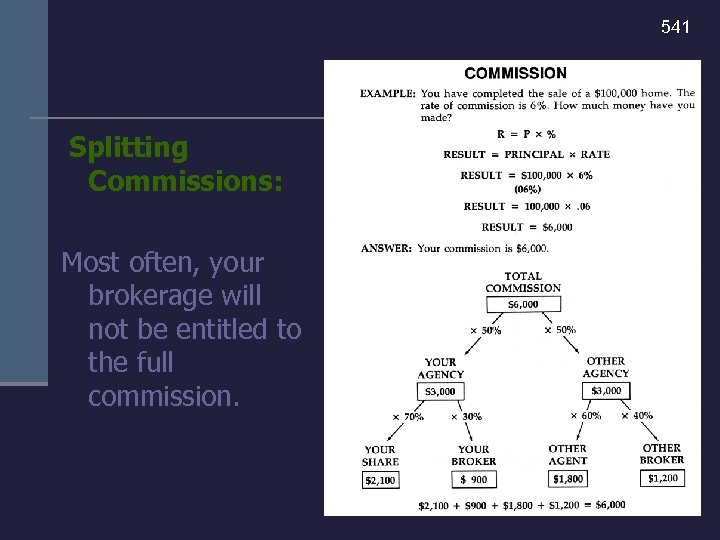541 Splitting Commissions: Most often, your brokerage will not be entitled to the full commission.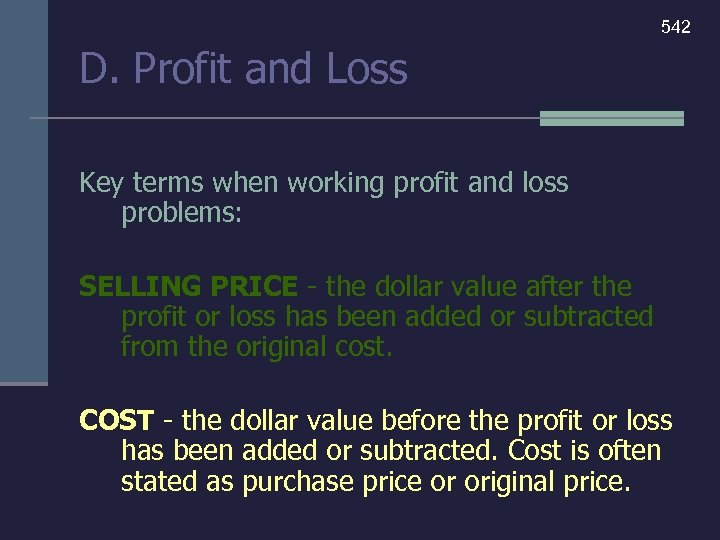542 D. Profit and Loss Key terms when working profit and loss problems: SELLING PRICE - the dollar value after the profit or loss has been added or subtracted from the original cost. COST - the dollar value before the profit or loss has been added or subtracted. Cost is often stated as purchase price or original price.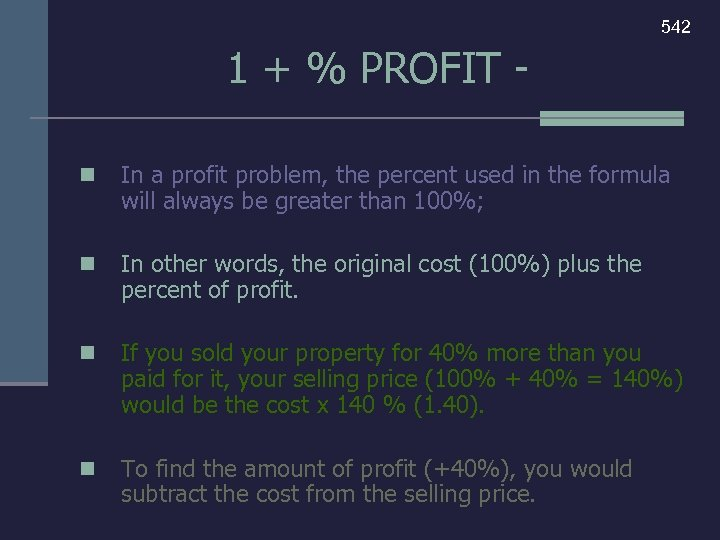542 1 + % PROFIT n In a profit problem, the percent used in the formula will always be greater than 100%; n In other words, the original cost (100%) plus the percent of profit. n If you sold your property for 40% more than you paid for it, your selling price (100% + 40% = 140%) would be the cost x 140 % (1. 40). n To find the amount of profit (+40%), you would subtract the cost from the selling price.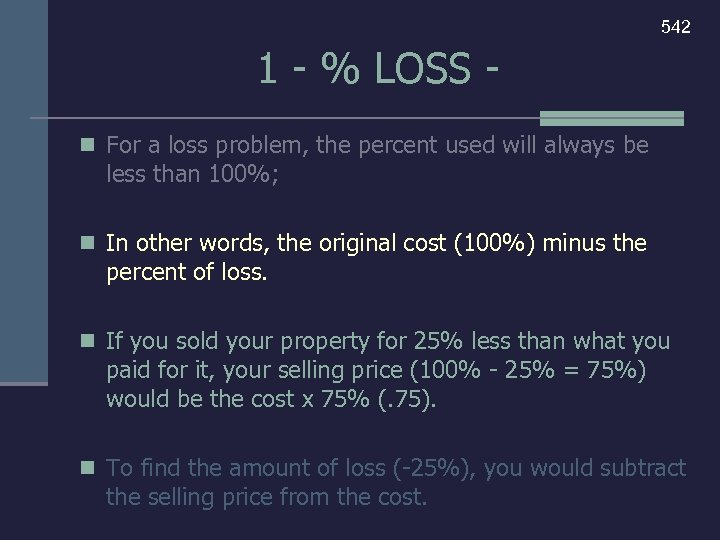542 1 - % LOSS n For a loss problem, the percent used will always be less than 100%; n In other words, the original cost (100%) minus the percent of loss. n If you sold your property for 25% less than what you paid for it, your selling price (100% - 25% = 75%) would be the cost x 75% (. 75). n To find the amount of loss (-25%), you would subtract the selling price from the cost.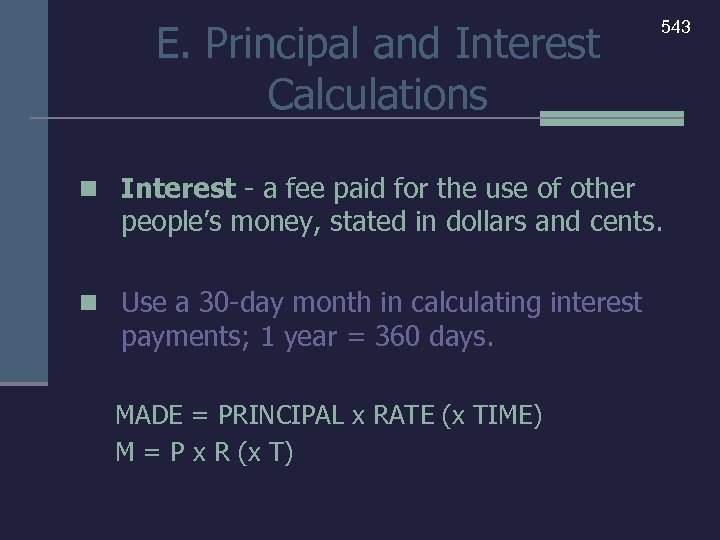E. Principal and Interest Calculations n Interest - a fee paid for the use of other 543 people’s money, stated in dollars and cents. n Use a 30 -day month in calculating interest payments; 1 year = 360 days. MADE = PRINCIPAL x RATE (x TIME) M = P x R (x T)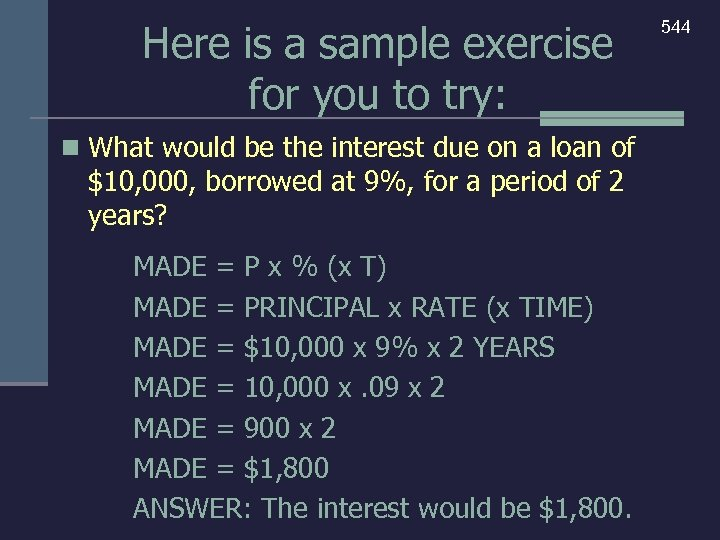Here is a sample exercise for you to try: n What would be the interest due on a loan of \$10, 000, borrowed at 9%, for a period of 2 years? MADE = P x % (x T) MADE = PRINCIPAL x RATE (x TIME) MADE = \$10, 000 x 9% x 2 YEARS MADE = 10, 000 x. 09 x 2 MADE = 900 x 2 MADE = \$1, 800 ANSWER: The interest would be \$1, 800. 544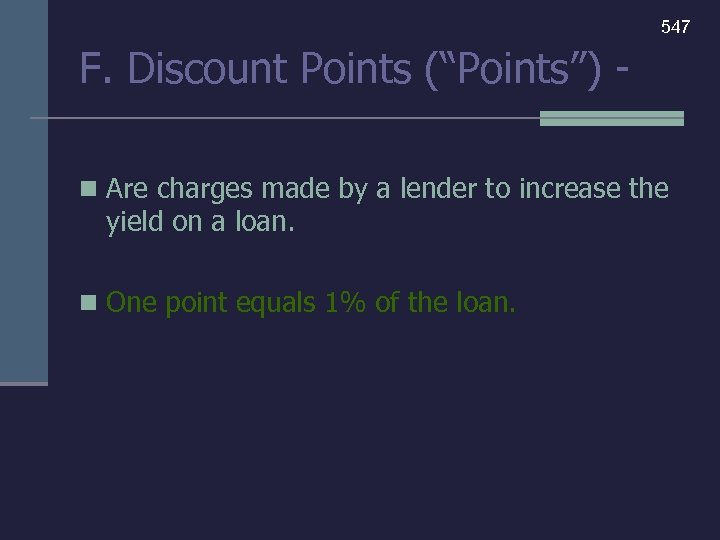547 F. Discount Points (“Points”) n Are charges made by a lender to increase the yield on a loan. n One point equals 1% of the loan.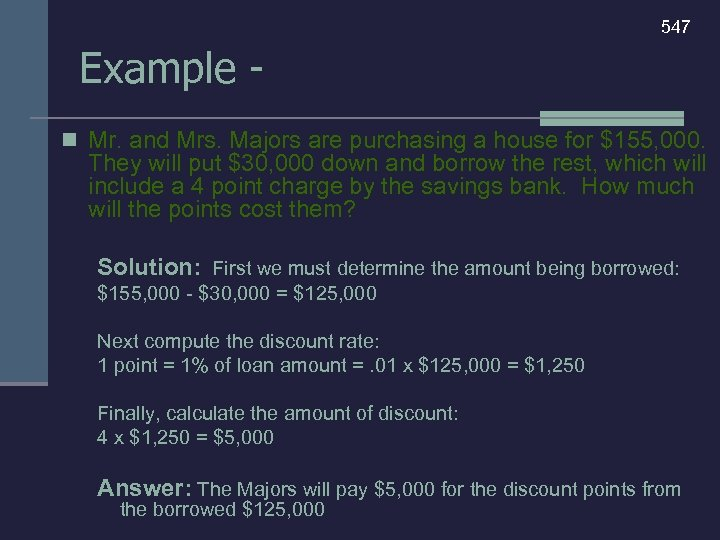547 Example n Mr. and Mrs. Majors are purchasing a house for \$155, 000. They will put \$30, 000 down and borrow the rest, which will include a 4 point charge by the savings bank. How much will the points cost them? Solution: First we must determine the amount being borrowed: \$155, 000 - \$30, 000 = \$125, 000 Next compute the discount rate: 1 point = 1% of loan amount =. 01 x \$125, 000 = \$1, 250 Finally, calculate the amount of discount: 4 x \$1, 250 = \$5, 000 Answer: The Majors will pay \$5, 000 for the discount points from the borrowed \$125, 000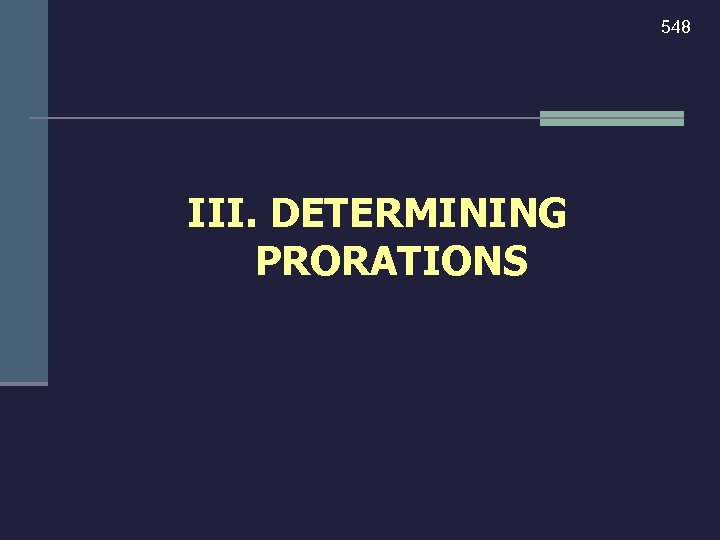548 III. DETERMINING PRORATIONS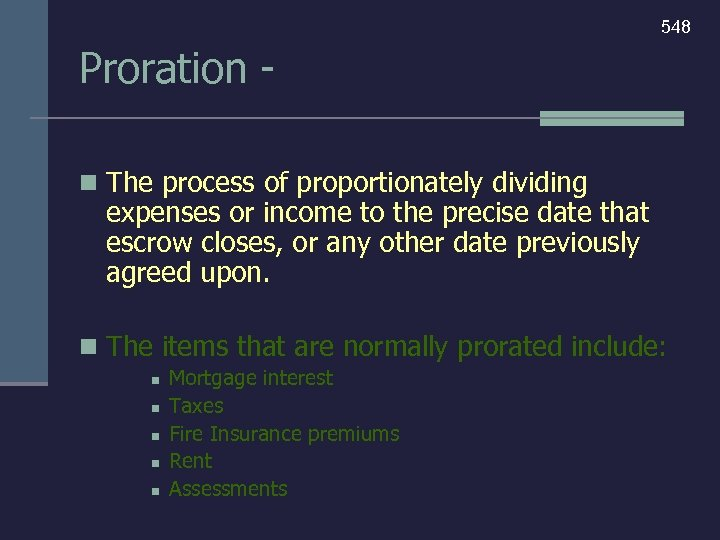548 Proration n The process of proportionately dividing expenses or income to the precise date that escrow closes, or any other date previously agreed upon. n The items that are normally prorated include: n n n Mortgage interest Taxes Fire Insurance premiums Rent Assessments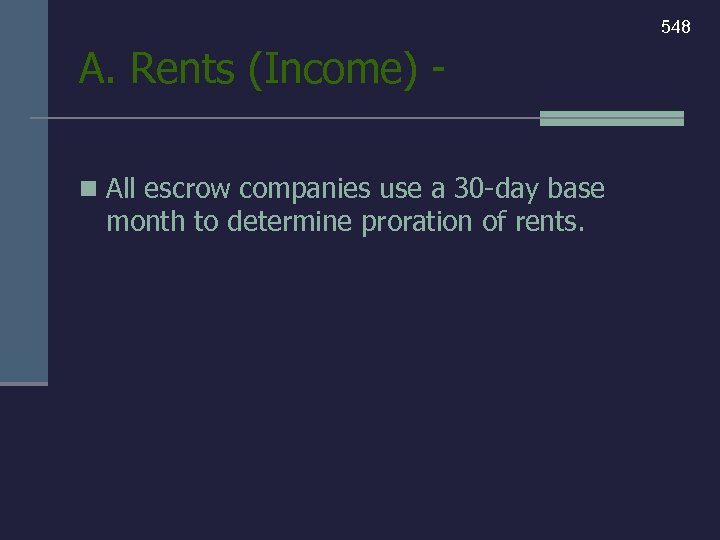548 A. Rents (Income) n All escrow companies use a 30 -day base month to determine proration of rents.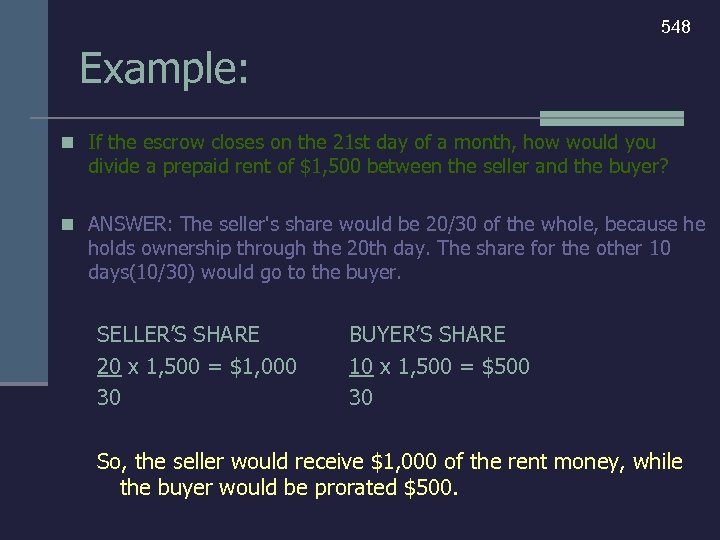548 Example: n If the escrow closes on the 21 st day of a month, how would you divide a prepaid rent of \$1, 500 between the seller and the buyer? n ANSWER: The seller's share would be 20/30 of the whole, because he holds ownership through the 20 th day. The share for the other 10 days(10/30) would go to the buyer. SELLER’S SHARE 20 x 1, 500 = \$1, 000 30 BUYER’S SHARE 10 x 1, 500 = \$500 30 So, the seller would receive \$1, 000 of the rent money, while the buyer would be prorated \$500.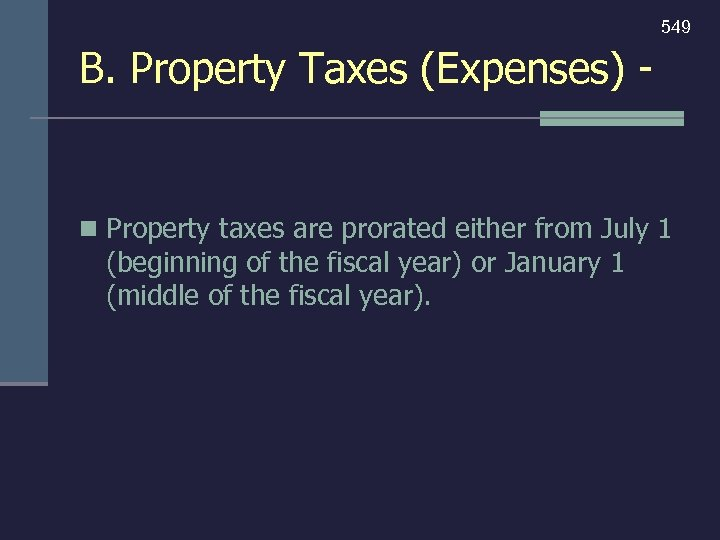549 B. Property Taxes (Expenses) - n Property taxes are prorated either from July 1 (beginning of the fiscal year) or January 1 (middle of the fiscal year).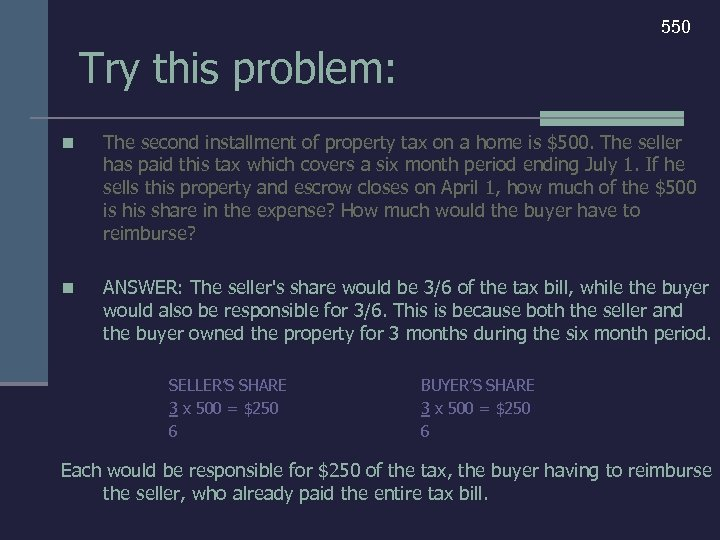550 Try this problem: n The second installment of property tax on a home is \$500. The seller has paid this tax which covers a six month period ending July 1. If he sells this property and escrow closes on April 1, how much of the \$500 is his share in the expense? How much would the buyer have to reimburse? n ANSWER: The seller's share would be 3/6 of the tax bill, while the buyer would also be responsible for 3/6. This is because both the seller and the buyer owned the property for 3 months during the six month period. SELLER’S SHARE 3 x 500 = \$250 6 BUYER’S SHARE 3 x 500 = \$250 6 Each would be responsible for \$250 of the tax, the buyer having to reimburse the seller, who already paid the entire tax bill.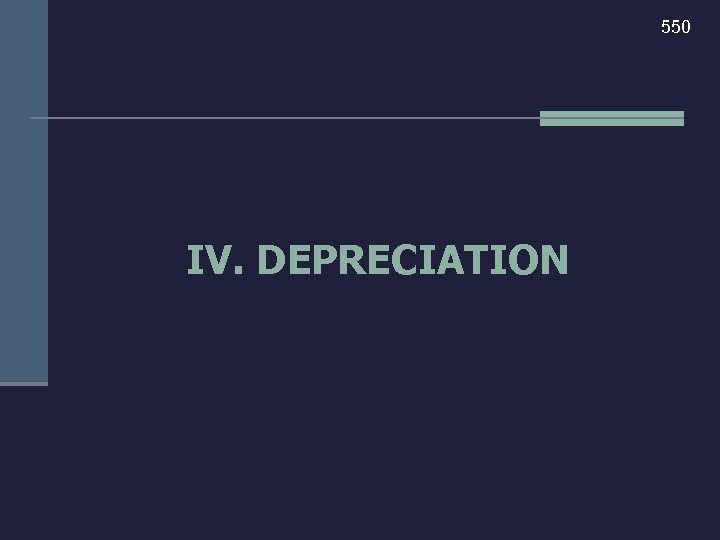550 IV. DEPRECIATION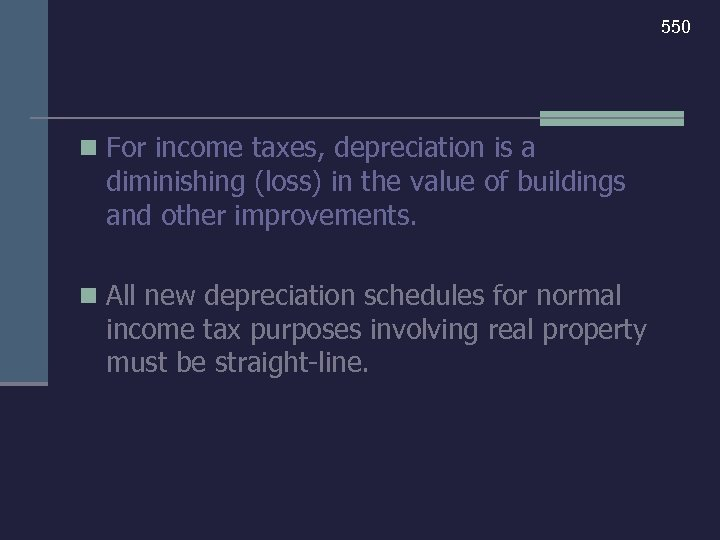550 n For income taxes, depreciation is a diminishing (loss) in the value of buildings and other improvements. n All new depreciation schedules for normal income tax purposes involving real property must be straight-line.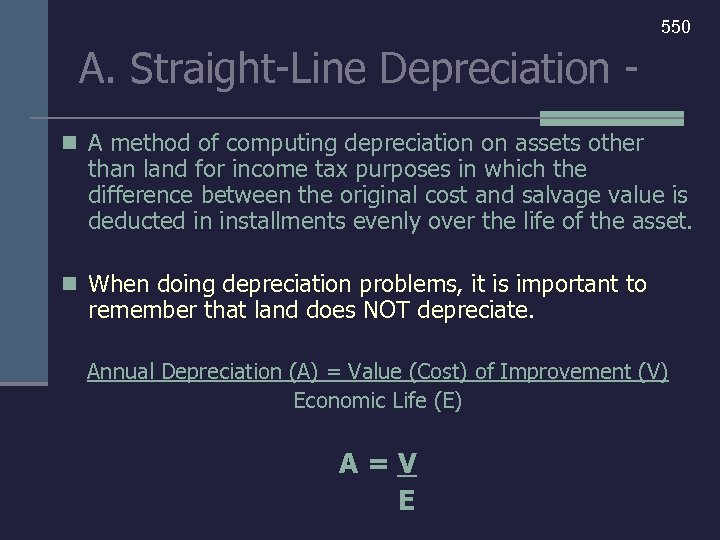550 A. Straight-Line Depreciation n A method of computing depreciation on assets other than land for income tax purposes in which the difference between the original cost and salvage value is deducted in installments evenly over the life of the asset. n When doing depreciation problems, it is important to remember that land does NOT depreciate. Annual Depreciation (A) = Value (Cost) of Improvement (V) Economic Life (E) A=V E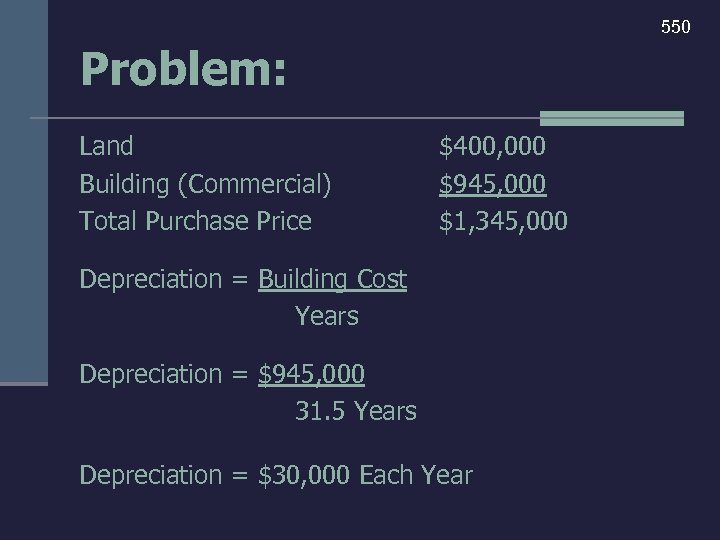550 Problem: Land Building (Commercial) Total Purchase Price \$400, 000 \$945, 000 \$1, 345, 000 Depreciation = Building Cost Years Depreciation = \$945, 000 31. 5 Years Depreciation = \$30, 000 Each Year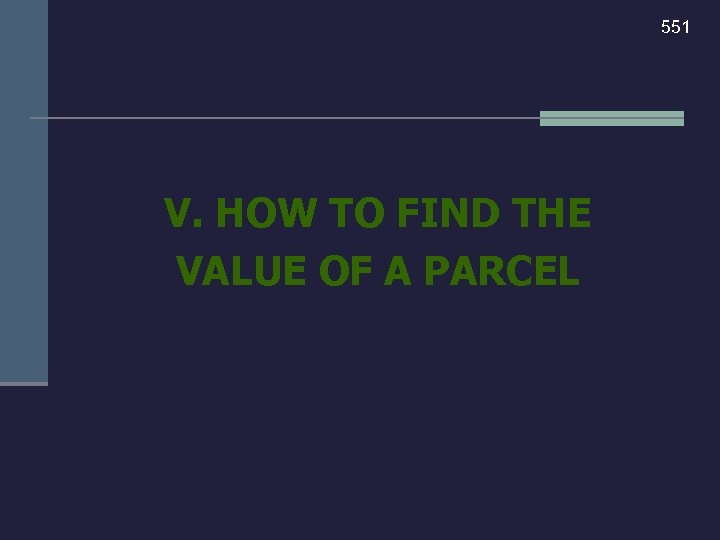551 V. HOW TO FIND THE VALUE OF A PARCEL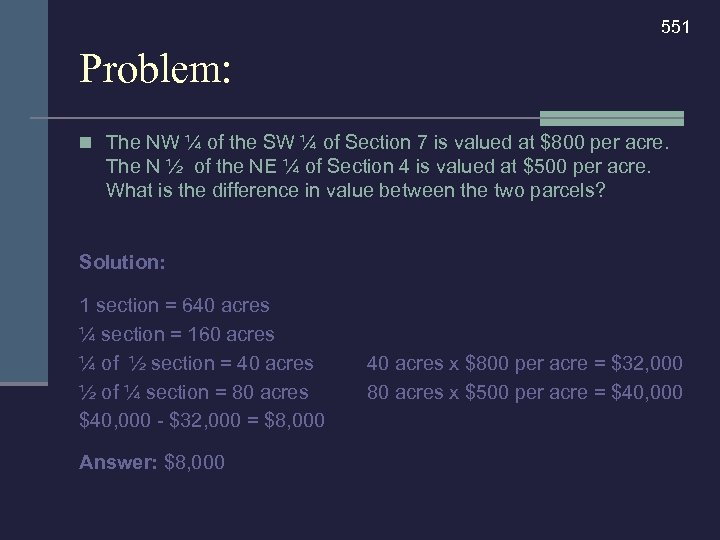551 Problem: n The NW ¼ of the SW ¼ of Section 7 is valued at \$800 per acre. The N ½ of the NE ¼ of Section 4 is valued at \$500 per acre. What is the difference in value between the two parcels? Solution: 1 section = 640 acres ¼ section = 160 acres ¼ of ½ section = 40 acres ½ of ¼ section = 80 acres \$40, 000 - \$32, 000 = \$8, 000 Answer: \$8, 000 40 acres x \$800 per acre = \$32, 000 80 acres x \$500 per acre = \$40, 000